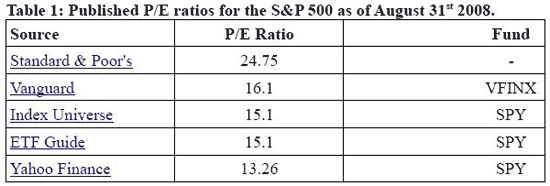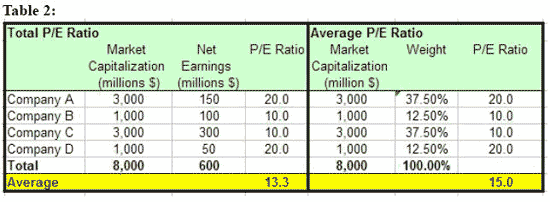# Has The U.S. Stock Market Become Cheaper?

October 05, 2008

Determining whether stocks have become more expensive or cheaper depends on which calculation method you use...

In the last 12 months, ending on September 30, 2008, the S&P 500 lost more than 20% of its value. Investors who are seeking opportunities in the current crisis are curious to know whether the U.S. stock market has become cheaper now. Surprisingly, a recent article in the New York Times (Why the bear is alive and well) claims that stocks have actually become more expensive. The reasoning is that the P/E ratio of the S&P 500 has gone up to almost 25—a very high value in historical perspective—according to Standard & Poor's calculations.

On the other hand, if you check any financial Web site that covers ETFs and you look for the P/E ratio of a fund that tracks the S&P 500, you will find a totally different story. In Table 1, I present some examples of P/E ratios that I collected from several financial data providers.The difference between the Standard & Poor's figure and the rest is striking, it's almost double in value. So whose figure is the correct one?

Apparently, the answer to that is not straightforward. The disparity in the figures is the result of two different methods of calculating the aggregate P/E ratio. The first method, which I will call the Total P/E Ratio, divides the total market value of all the companies in the index by their total net earnings. This method is used by Standard & Poor's. The second method, which is called the Average P/E Ratio, calculates the aggregate ratio as the weighted average of the P/E ratios of all the companies in the index. This method is used by all the other financial data providers.

An illustration of the two methods is presented in Table 2. For illustrative purposes, I have constructed a synthetic index that comprises four companies: large-cap with high P/E ratio; small-cap with high P/E ratio; large-cap with low P/E ratio and small-cap with low P/E ratio. The calculation of the aggregate P/E ratio by each method is presented in the last (yellow) row.The aggregate P/E ratios that are calculated by each method are not identical, but they are quite similar and do not provide an explanation to the disparity shown in Table 1. The difference between the methods arises when one (or more) of the companies is reporting a net loss. At the company level, the P/E ratio has no meaning when the bottom line is negative, and thus it is not calculated. At the index level, however, the treatment of losses makes all the difference between the two methods. The Total P/E Ratio approach is straightforward: The numerator of the ratio is the total market value and the denominator is the total net earnings; losses are counted as net earnings with a minus sign, and thus decrease the denominator and increase the P/E ratio.

How does the Average P/E Ratio approach address losses? None of the financial data providers gives any details about this issue. Nevertheless, the most plausible way is to exclude the companies with the losses and then to recalculate the weights of the index only for the companies that have a valid P/E ratio. Based on these new weights, the Average P/E can be calculated.

Each one of the methods has its pros and cons. The Total P/E Ratio looks at the index as one company with many profit centers. If this were the case, it is obviously the best method. However, an index is not equivalent to a large company. P/E ratios of loss-making companies are not calculated because they are not economically meaningful. If investors had thought that a company would continue to lose money in the long run, its market value would have become zero. Thus, it is more reasonable to assume that investors relate to its losses as a temporary phenomenon and use a hypothetical profit in order to determine its value. In that respect, it makes sense to exclude such company from the calculation of the average P/E.

Providing that the weight of the excluded companies is only a small fraction of the index, the Average P/E Ratio can be more informative. In order to illustrate this point, I have analyzed the behavior of the two aggregate P/E ratios using the synthetic index of Table 2. In the first stage, I calculated the aggregate P/E ratios using four scenarios. In each one of them, one of the companies is reporting a net loss. I kept the values of all the other variables unchanged in order to measure only the effect of the reported net loss. The calculation of the P/E ratios in the four scenarios is presented in Table 3.